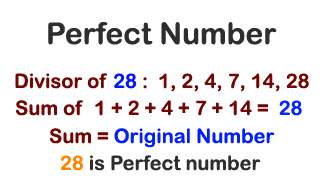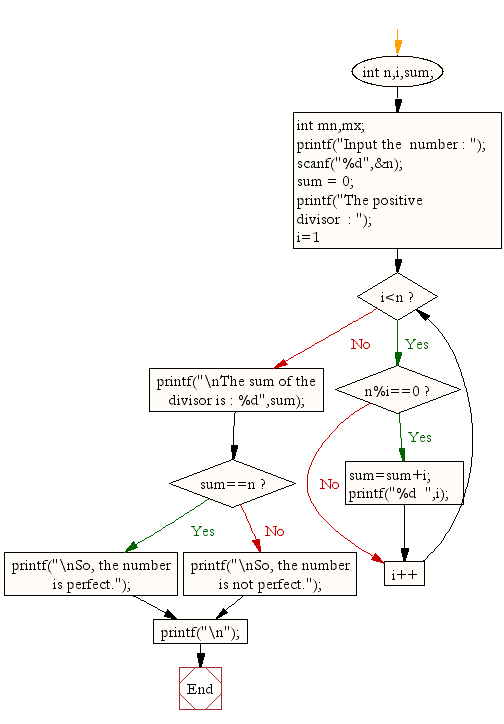﻿ C : Check whether a given number is a perfect number or not# C Exercises: Check whether a given number is a perfect number or not

## C For Loop: Exercise-27 with Solution

Write a c program to check whether a given number is a perfect number or not.

Pictorial Presentation:Sample Solution:

C Code:

``````/*Perfect number is a positive number which sum of all positive divisors excluding that number is equal to that number. For example 6 is perfect number since divisor of 6 are 1, 2 and 3.  Sum of its divisor is 1 + 2+ 3 = 6*/
#include <stdio.h>

void  main()
{
int n,i,sum;
int mn,mx;

printf("Input the  number : ");
scanf("%d",&n);
sum = 0;
printf("The positive divisor  : ");
for (i=1;i<n;i++)
{
if(n%i==0)
{
sum=sum+i;
printf("%d  ",i);
}
}
printf("\nThe sum of the divisor is : %d",sum);
if(sum==n)
printf("\nSo, the number is perfect.");
else
printf("\nSo, the number is not perfect.");
printf("\n");
}
```
```

Sample Output:

```Input the  number : 56
The positive divisor  : 1  2  4  7  8  14  28
The sum of the divisor is : 64
So, the number is not perfect.
```

Flowchart:C Programming Code Editor:

Improve this sample solution and post your code through Disqus.

What is the difficulty level of this exercise?

Test your Programming skills with w3resource's quiz.

﻿

## C Programming: Tips of the Day

What is the size of an enum in c?

An enum is only guaranteed to be large enough to hold int values. The compiler is free to choose the actual type used based on the enumeration constants defined so it can choose a smaller type if it can represent the values you define. If you need enumeration constants that don't fit into an int you will need to use compiler-specific extensions to do so.

Ref : https://bit.ly/3ypg4k8